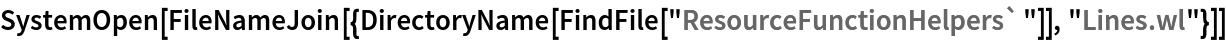Function Repository Resource:

# TangentAndNormalLine

Compute properties of the tangent and normal lines to a curve at a given point

Contributed by: Wolfram|Alpha Math Team
 ResourceFunction["TangentAndNormalLine"][expr,{x,a},{y,b}] gives an association of properties of the tangent and normal lines to expr, viewed as an equation in x and y, at the point {x,y}={a,b}. ResourceFunction["TangentAndNormalLine"][expr,{x,a},{y,b},prop] returns the value of the tangent and normal lines property prop. ResourceFunction["TangentAndNormalLine"][expr,{x,a},y] returns information relating to one pair, among possibly several, of the tangent and normal lines to expr at x=a. ResourceFunction["TangentAndNormalLine"][expr,x,{y,b}] returns information relating to one pair, among possibly several, of the tangent and normal lines to expr at y=b.

## Details

Allowed values of prop are:
 "SlopeInterceptEquation" equation of the tangent line in slope intercept form "StandardFormEquation" equation of the tangent line in standard form "PointSlopeEquation" equation of the tangent line in point slope form "HorizontalIntercept" horizontal intercept for the tangent line equation "VerticalIntercept" vertical intercept for the tangent line equation "Plot" plot of the tangent line equation All association of information returning all allowed properties
If expr does not have head Equal, then expr is treated as an expression defining y in terms of x. In other words, ResourceFunction["TangentAndNormalLine"][expr,{x,a},y,] is equivalent to ResourceFunction["TangentAndNormalLine"][y==expr,{x,a},y,] if expr has a head other than Equal.
If only one coordinate of the intersection point is given, the other coordinate is inferred. For expressions that are multivalued at the given value of x or y, information on only one of potentially several tangent and normal line pairs is returned.

## Examples

### Basic Examples (2)

Compute the slope-intercept equations of the tangent and normal lines to a curve at a given point:

 In:=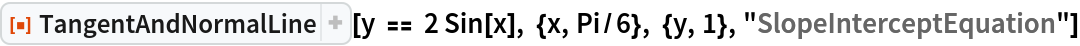Out=Visualize this result:

 In:=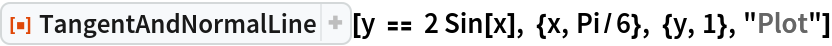Out=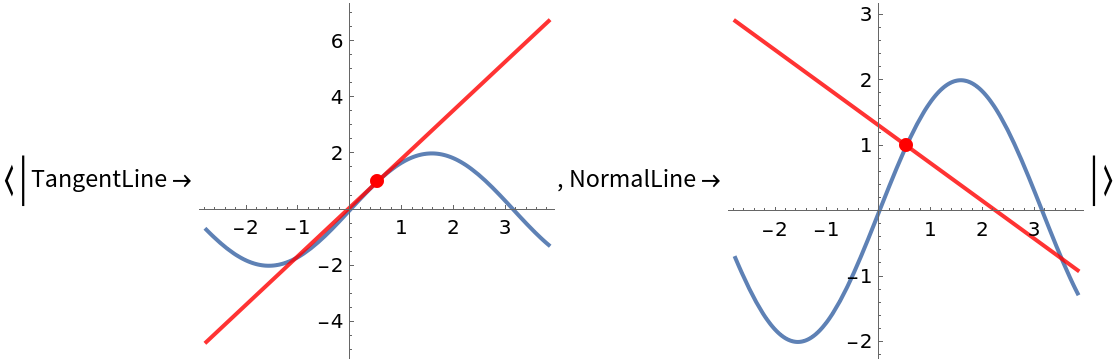Compute the slope of these tangent and normal lines:

 In:=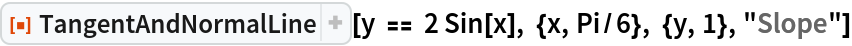Out=Compute the horizontal intercepts of these tangent and normal lines:

 In:=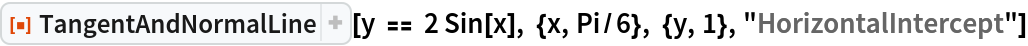Out=Get the standard-form equation of these tangent and normal lines:

 In:=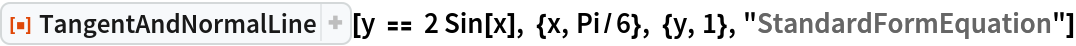Out=Get an association of properties of the tangent and normal lines to a curve:

 In:=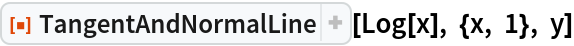Out=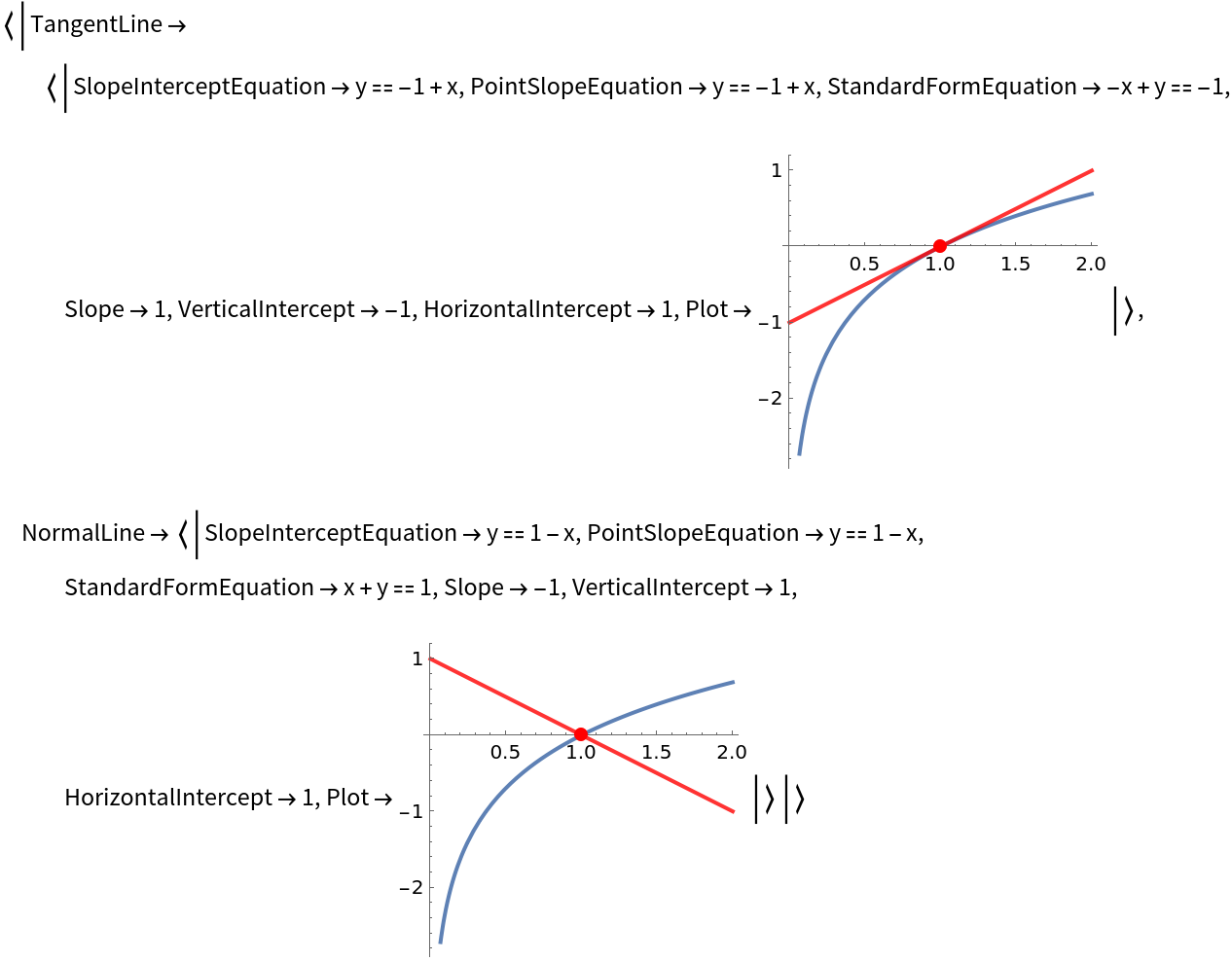Get just the point-slope equations:

 In:=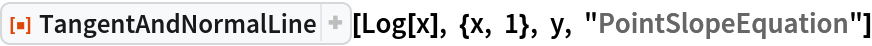Out=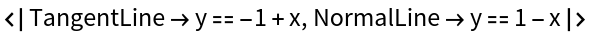### Scope (1)

The first argument to TangentAndNormalLine can be an implicit definition of a curve:

 In:=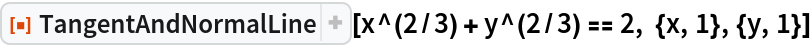Out=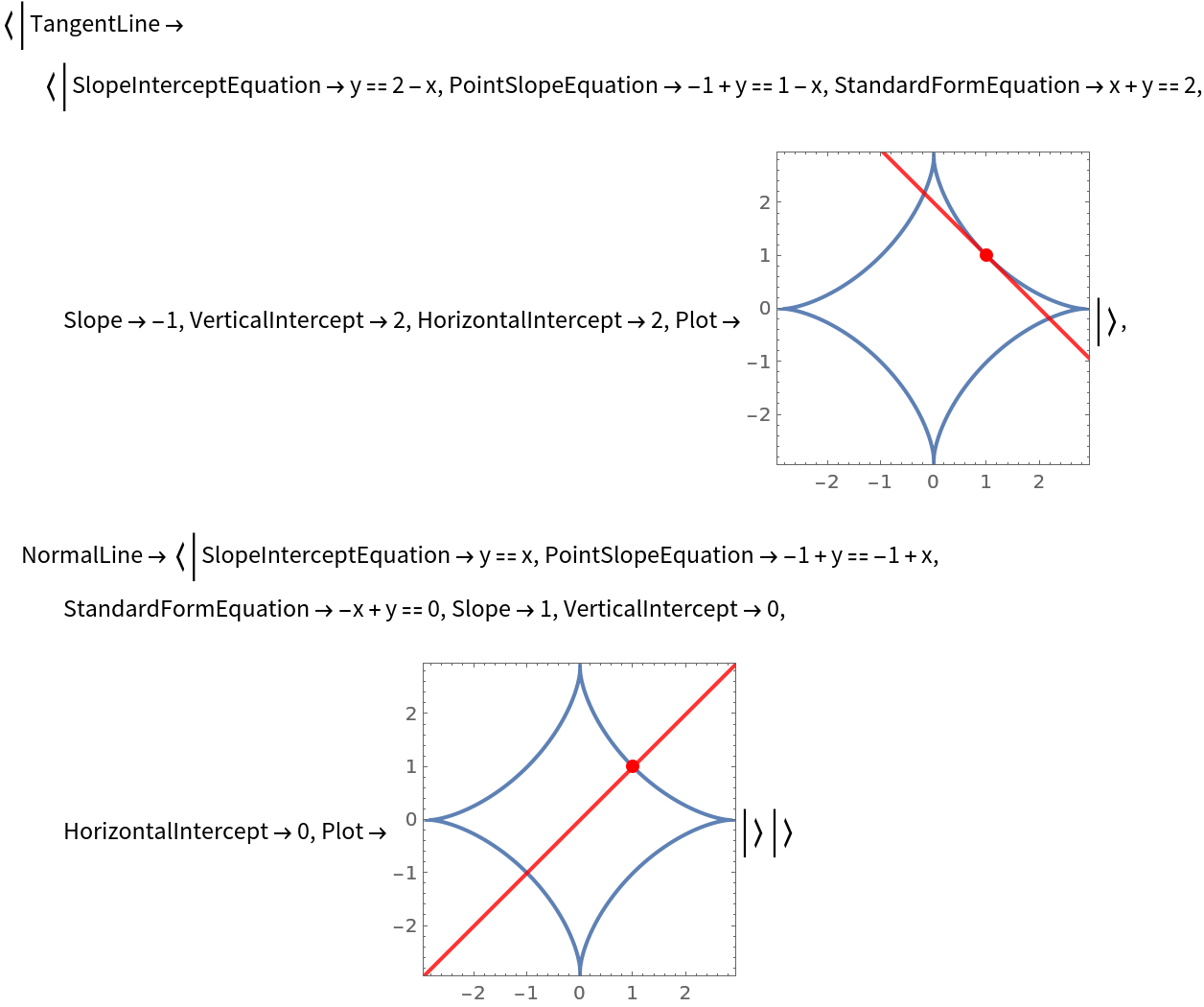### Properties and Relations (1)

If a tangent or normal line is parallel to a coordinate axis, its intercept with that axis is None:

 In:=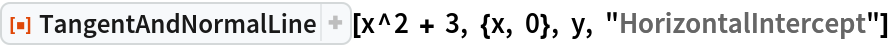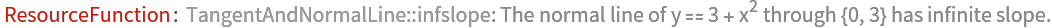Out=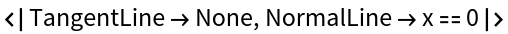### Possible Issues (3)

If a position for y is not specified, information on only one of the possible normal lines at the given x value is returned:

 In:=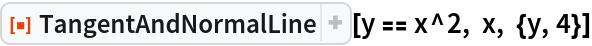Out=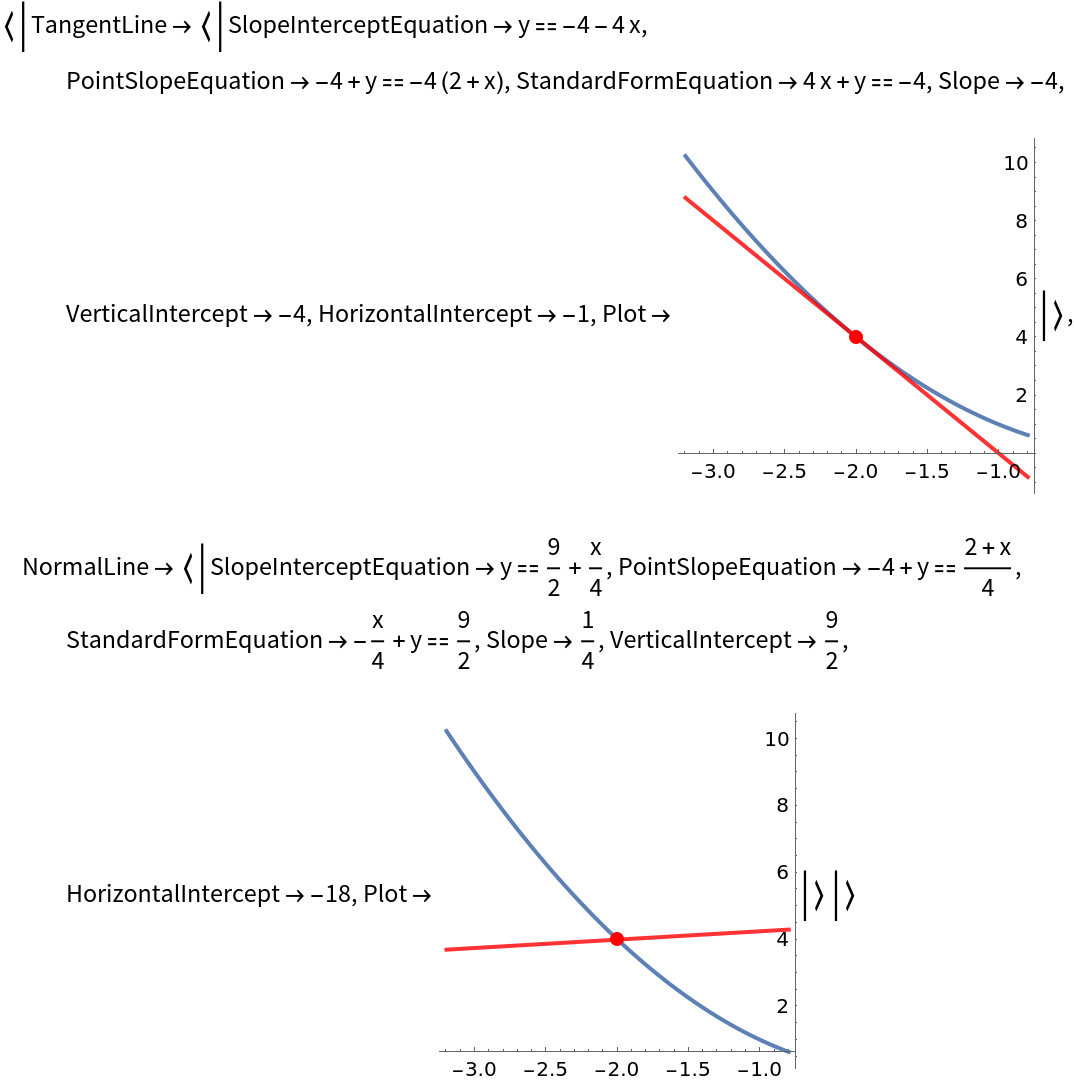Requesting tangent and normal lines information about a point that is not on the curve will result in an error message:

 In:=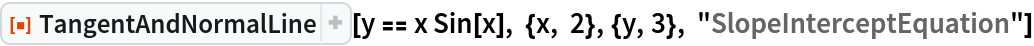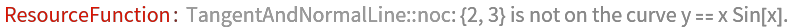Out=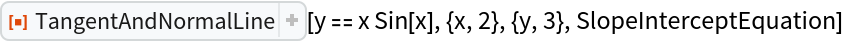Vertical tangent lines (whose slope cannot be computed) are plotted as dotted lines. Some of their properties may not be defined:

 In:=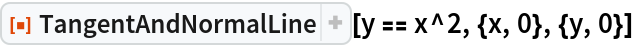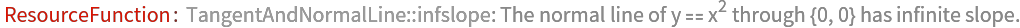Out=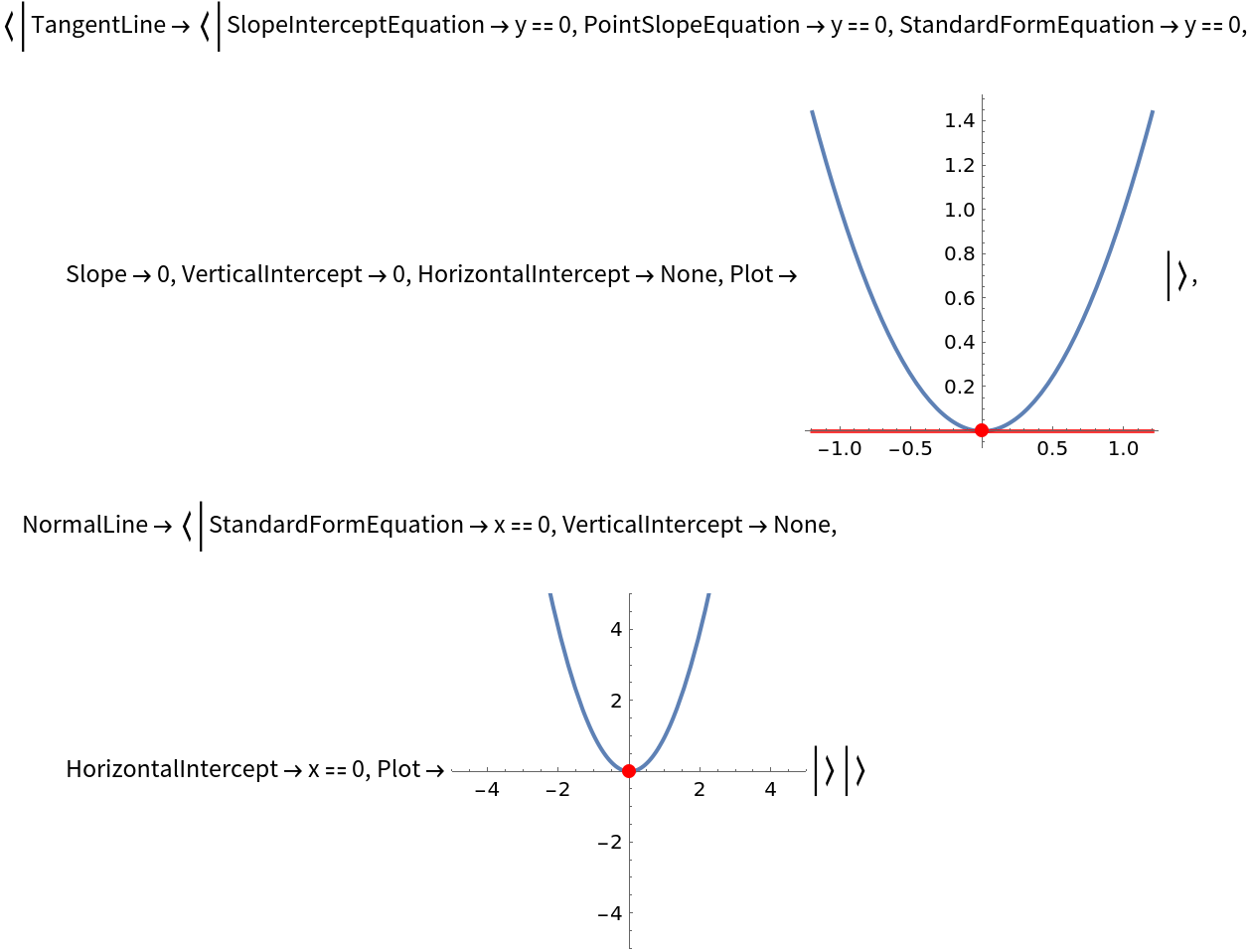If a function has a cusp or a discontinuity at the given point, no tangent or normal line is returned:

 In:=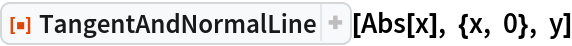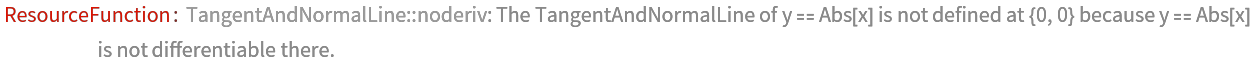Out=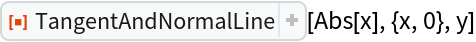## Publisher

Wolfram|Alpha Math Team

## Version History

• 2.0.0 – 23 March 2023
• 1.0.0 – 15 June 2021

## Author Notes

To view the full source code for TangentAndNormalLine, evaluate the following:

 In:=